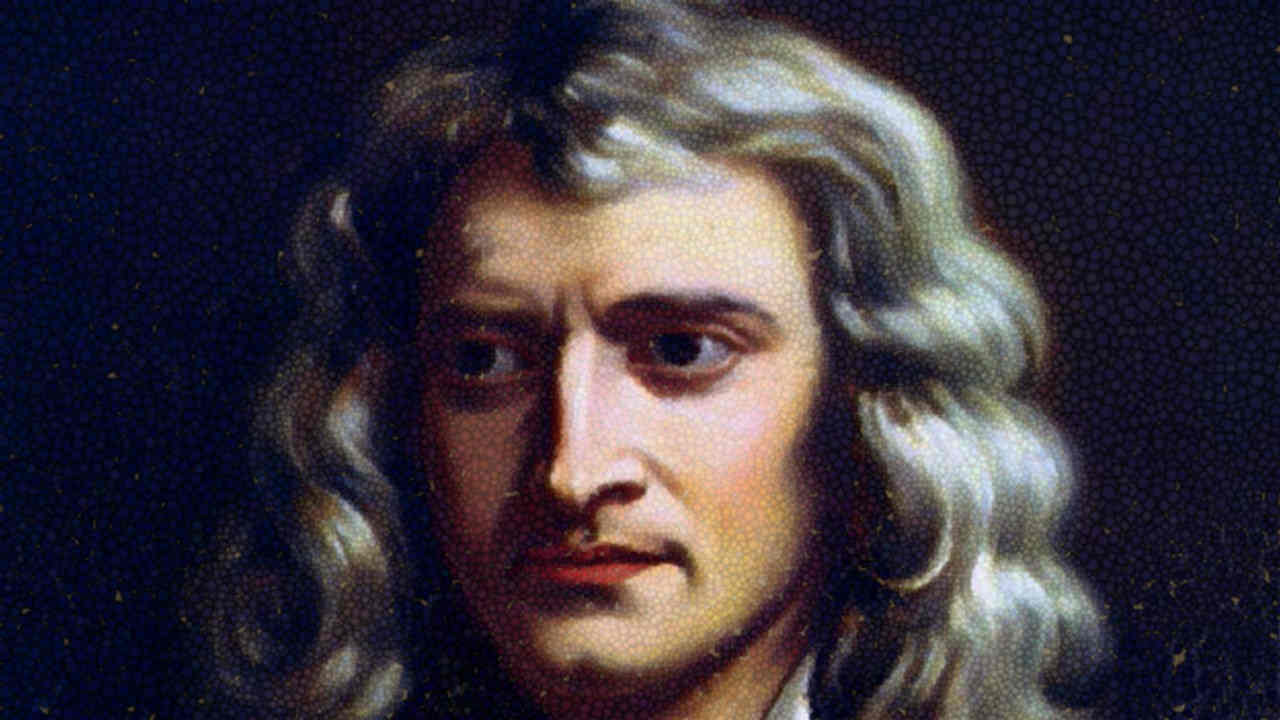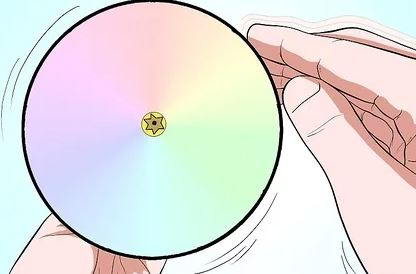# 10 Discoveries By Newton That Changed The WorldIsaac Newton is one of the few names that will forever be enshrined in physics history and that too with a lot of glamour associated. Contributions of none other physicist match his, well, probably Einstein's, or not even his!? The following are Newton's ten most well-known works that changed the world later on.#### Laws of motion

1. An object will remain at rest or move in a straight line unless acted upon by an external force.
3. For every action, there is an equal and opposite reaction.

Newton's three laws of motion, along with thermodynamics, stimulated the industrial revolution of the 18th and 19th centuries. Much of the society built today owes to these laws.

#### Binomial Theorem

Around 1665, Isaac Newton discovered the Binomial Theorem, a method to expand the powers of sum of two terms. He generalized the same in 1676. The binomial theorem is used in probability theory and in the computing sciences.

#### Inverse square law

By using Kepler's laws of planetary motion, Newton derived the inverse square law of gravity. This means that the force of gravity between two objects is inversely proportional to the square of the distance between their centers. This law is used to launch satellites into space.

#### Newton's cannon

Newton was a strong supporter of Copernican Heliocentrism. This was a thought experiment by Newton to illustrate orbit or revolution of moon around earth (and hence, earth around the Sun).He imagined a very tall mountain at the top of Earth on which a cannon is loaded. If too much gunpowder is used, then the cannonball will fly into space. If too little is used, then the ball wouldn't travel far. Just the right amount of powder will make the ball orbit the Earth.

#### Calculus

Newton invented the differential calculus when he was trying to figure out the problem of accelerating body. Whereas Leibniz is best-known for the creation of integral calculus. The calculus is at the foundation of higher level mathematics. Calculus is used in physics and engineering, such as to improve the architecture of buildings and bridges.

#### Rainbow

Newton was the first to understand the formation of rainbow. He also figured out that white light was a combination of 7 colors. This he demonstrated by using a disc, which is painted in the colors, fixed on an axis. When rotated, the colors mix, leading to a whitish hue.Newton's disc

#### Reflecting Telescope

In 1666, Newton imagined a telescope with mirrors which he finished making two years later in 1668. It has many advantages over refracting telescope such as clearer image, cheap cost, etc.

#### Law of cooling

His law states that the rate of heat loss in a body is proportional to the difference in the temperatures between the body and its surroundings. The more the difference, the sooner the cup of tea will cool down.

#### Classification of cubics

Newton found 72 of the 78 "species" of cubic curves and categorized them into four types. In 1717, Scottish mathematician James Stirling proved that every cubic was one of these four types.some cubic curves (Wiki)

#### Alchemy

At that time, alchemy was the equivalent of chemistry. Newton was very interested in this field apart from his works in physics. He conducted many experiments in chemistry and made notes on creating a philosopher's stone.

Newton could not succeed in this attempt but he did manage to invent many types of alloys including a purple copper alloy and a fusible alloy (Bi, Pb, Sn). The alloy has medical applications (radiotherapy).